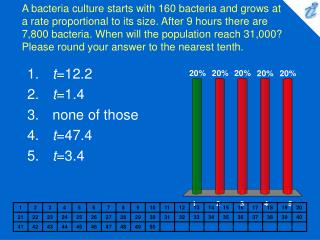DownloadDownload Presentationt =12.2 t =1.4 none of those t =47.4 t =3.4

# t =12.2 t =1.4 none of those t =47.4 t =3.4

Download Presentation## t =12.2 t =1.4 none of those t =47.4 t =3.4

- - - - - - - - - - - - - - - - - - - - - - - - - - - E N D - - - - - - - - - - - - - - - - - - - - - - - - - - -
##### Presentation Transcript

1. A bacteria culture starts with 160 bacteria and grows at a rate proportional to its size. After 9 hours there are 7,800 bacteria. When will the population reach 31,000? Please round your answer to the nearest tenth. • t=12.2 • t=1.4 • none of those • t=47.4 • t=3.4

2. Experiments show that if the chemical reaction {image} takes place at 45 {image} C, the rate of reaction of dinitrogen pentoxide is proportional to its concentration as follows: {image} How long will the reaction take to reduce the concentration of {image} to 30% of its original value? • t = 1,204 • none of those • t = 1,166 • t = 2,108 • t = 1,018 • t = 1,338

3. 1. 2. 3. 4. 5. 6. A curve passes through the point ( 9, 9 ) and has the property that the slope of the curve at every point P is 3 times the y-coordinate P. What is the equation of the curve? • {image} • none of those • {image} • {image} • {image} • {image}

4. The rate of change of atmospheric pressure P with respect to altitude h is proportional to P provided that the temperature is constant. At 15 {image} C the pressure is 100.6 kPa at sea level and 89.4 kPa at h = 910. What is the pressure at an altitude of 3,800 m? • P = 27.9 kPa • P = 58.8 kPa • P = 66.8 kPa • P = 61.5 kPa • P = 104.5 kPa • none of those

5. Consider a population P = P(t) with constant relative birth and death rates {image} and {image} , respectively, and a constant emigration rate m, where {image} and m = 0.7. Then the rate of change of the population at time t is modeled by the differential equation {image} where {image} . Find the solution of this equation with the rate of change of the population at time t = 3 that satisfies the initial condition P(0) = 3,000. • 13,440.2 • none of those • 16,440.2 • 1,920.0 • 13,449.2 • 10,440.2